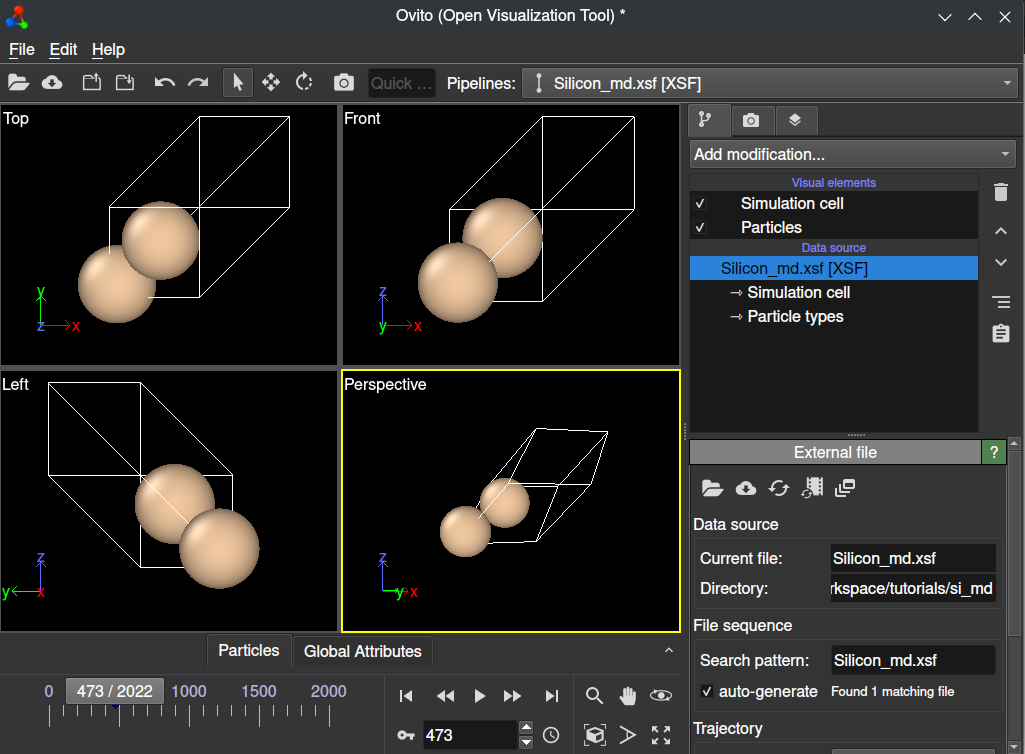# Silicon AIMD

We will show an ab-initio molecular dynamics simulation with a silicon crystal using QimPy. Ab-initio molecular dynamics (AIMD) is a technique for performing molecular dynamics simulations using electronic structure calculations to compute forces. Although AIMD is significantly more computationally intensive than conventional molecular dynamics, you should find that the calculation in this tutorial runs on ordinary consumer hardware, with significant benefits to GPU-based computation if it is available.

The following input file defines an AIMD calculation on a perturbed diamond-cubic silicon lattice with a Nose-Hoover thermostat. Save this text to `Silicon_md.yaml` in your calculation directory:

```# Diamond-cubic silicon
lattice:
system: cubic
modification: face-centered
a: 5.4437022209 Angstrom
movable: no

ions:
pseudopotentials:
- SG15/\$ID_ONCV_PBE-1.0.upf
coordinates:
- [Si, 0.75,   0.75,   0.25]
- [Si, 0.00,   0.50,   0.50]
- [Si, 0.75,   0.25,   0.75]
- [Si, 0.00,   0.00,   0.00]
- [Si, 0.25,   0.75,   0.75]
- [Si, 0.50,   0.50,   0.00]
- [Si, 0.25,   0.25,   0.25]
- [Si, 0.50,   0.00,   0.50]

geometry:
dynamics:
T0: 300 K
n-steps: 5000
dt: 10. fs
thermostat:
nose-hoover:
chain-length-T: 3
t-damp-T: 50 fs

checkpoint: null  # disable reading checkpoint
checkpoint-out: Silicon_md.h5  # but still create it
```

Now you are ready to perform the calculation:

```(qimpy) \$ python -m qimpy.dft -i Silicon_md.yaml | tee Silicon_md.out
```

The standard output of this run will be saved to `Silicon_md.out`, and raw data (including forces, trajectories, and all run parameters) will be saved to `Silicon_md.h5` (as instructed by `checkpoint-out`). If this calculation ends and you would like to restart or continue it from a checkpoint, simply change `checkpoint: null` to `checkpoint: Silicon_md.h5`. The trajectories from this new run will be seamlessly appended to the existing checkpoint file.

```import h5py
import matplotlib.pyplot as plt
import numpy as np

silicon_md = h5py.File("Silicon_md.h5", "r")

# Energy per step
E = np.array(silicon_md["geometry"]["action"]["history"]["energy"])

dt = 10. # fs
steps = len(E)
time = np.linspace(0, steps*dt, num=steps)

# Temperature per step (as an example)
T = np.array(silicon_md["geometry"]["action"]["history"]["T"])

# Make a time series plot
plt.ylabel("Energy vs. Time (Silicon AIMD)")
plt.ylabel("Energy (Ha)")
plt.xlabel("Time (fs)")
plt.plot(time, E)

plt.savefig("si_aimd_energy.png")
```

Save this script as `energy_plot.py` within your calculation directory (make sure the `Silicon_md.h5` checkpoint file is available), and run it to produce the following time-series plot of the system’s energy:

You may just as easily extract all other time series parameters of your run as numpy arrays for analysis (e.g. forces, positions, etc.).

Using QimPy’s XSF interface, you can also easily extract this data to create an animated XSF file for analysis with standard tools such as Ovito. You can do this by running the following script within your calculation directory:

```(qimpy) \$ python -m qimpy.interfaces.xsf --animated -c Silicon_md.h5 -x Silicon_md.xsf
```

The `--animated` flag makes sure that this data is parsed into an animated XSF file. You may now open this file in Ovito, and you will be able to view an animation of your calculation.